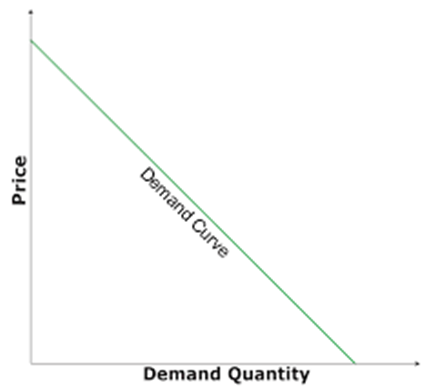Economics (NCERT) Notes → Class XII

2.15 Relationship between Elasticity and Expenditure

Elasticity and Expenditure•The expenditure on a good is equal to the (demand for the good)x(price)
•The price of a good and the demand for the good are inversely related to each other.
•Whether the expenditure on the good goes up or down as a result of an increase in its price depends on how responsive the demand for the good is to the price change.

Effect of Increase in Price
•If the percentage decline in quantity is greater than the percentage increase in the price, the expenditure on the good will go down.
•If the percentage decline in quantity is less than the percentage increase in the price, the expenditure on the good will go up
•If the percentage decline in quantity is equal to the percentage increase in the price, the expenditure on the good will remain unchanged

Effect of decline in the price
•If the percentage increase in quantity is greater than the percentage decline in the price, the expenditure on the good will go up
•If the percentage increase in quantity is less than the percentage decline in the price, the expenditure on the good will go
•If the percentage increase in quantity is equal to the percentage decline in the price, the expenditure on the good will remain unchanged

Expenditure and Elasticity
•The expenditure on the good would change in the opposite direction as the price change if and only if the percentage change in quantity is greater than the percentage change in price, i.e. if the good is price-elastic.
•The expenditure on the good would change in the same direction as the price change if and only if the percentage change in quantity is less than the percentage change in price, i.e., if the good is price inelastic
•The expenditure on the good would remain unchanged if and only if the percentage change in quantity is equal to the percentage change in price, i.e., if the good is unit-elastic

Relationship between Elasticity and Expenditure
•Suppose
•at price p, the demand for a good is q, and
•at price p + ∆ p, the demand for the good is q + ∆ q.
•Expenditure
•At price p, the total expenditure on the good is pq, and
•At price p + ∆ p, the total expenditure on the good is (p + ∆ p)(q + ∆ q).
•If price changes from p to (p + ∆ p),
•the change in the expenditure on the good (∆ E )
= (p + ∆ p)(q + ∆ q) – pq
= qp + pq + ∆ pq
=qp + pq (For small values of ∆ p and ∆ q)
= p q[1 +p/q(q/ ∆ p)]
=p q[1 +eD)]

Relationship between Elasticity and Expenditure
•If price changes from p to (p + ∆ p), the change in the expenditure on the good ∆ E =p q[1 +eD)]
•if eD < –1 (elastic), then q (1 + eD) < 0, and hence, ∆ E has the opposite sign as ∆ p,
•if eD > –1 (inelastic), then q (1 + eD) > 0, and hence, ∆ E has the same sign as ∆ p,
•if eD = 1 (unit-elastic), then q (1 + eD) = 0, and hence, ∆ E = 0.

 Related Articles
 • 3.2 Inequality in Poverty • 2.3 The Problem of Unemployment • 2.2 Quality of Population • 2.1 People as Resource • 1.2 Increasing Agricultural Productivity • 5.4 Case Studies of Consumer Rights • 5.3 Consumer Protection Act • 5.1 Consumer Rights • 4.4 Impact of Globalization in India • 4.2 Foreign Trade and Integration of Markets
 Recent Articles
 • “Poverty anywhere is a threat to prosperity everywhere”  • GDP along with GNH as the indices for measuring progress • Nobel Peace Prize, 2020 • Convention on the conservation of migratory species of wild animals India • Emerging Technologies Division set up • Decade of Healthy Ageing • The relevance of Gandhi in modern times  • Hyper Globalism: a threat to human prosperity? • Business Process Outsourcing in India: a booming phenomenon?  • Covid-19 and the BioMedical Waste crisis